Worksheet Question: Practical Geometry

# Worksheet Question: Practical Geometry - Notes | Study Mathematics (Maths) Class 6 - Class 6

 1 Crore+ students have signed up on EduRev. Have you?

Q.1. Choose suitable units for the following measurements :
(a) Lenght of your pencil (cm or km) cm _(_fo_r_ e_x_a_m__p_le)
(b) Your height (cm or km) __________
(c) Distance by road between Delhi and Jaipur (cm or km) __________
(d) Lenght of your feet (cm or km) __________
(e) Length of rice grain (mm or km) __________
(f) Length of cloth needed to make your long raincoat (m or km) __________

Ans.
(a) cm
(b) cm
(c) km
(d) cm
(e) mm
(f) m

Q.2. In the figure, OA = AB = BC = CD Fill in the blanks :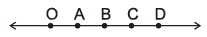(i) A is the mid point of line segment __________.
(ii) B is the mid point of line segment __________ and line segment __________ also.
(iii) OC + CD = __________ (iv) OC – OA = __________
(v) Name the line segment whose length is equal to 3 times OA.

Ans.
(i) OB
(ii) AC and OD
(iii) OD
(v) AC
(v) OC

Q.3. Which angle is bigger? ∠ABC or ∠DEF?

Ans. Both angle, are equal, ∠ABC = ∠DEF

Q.4. Using ruler and compass to construct angles of following measures :
(a) 135°
(b) 90°
(c) 180°
(d) 15°

Ans. Angle construction.

Q.5. Draw an angle measuring 54° using protractor. Now, without using protractor, construct
(a) a copy of this angle.
(b) an angle measuring 27°.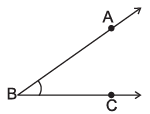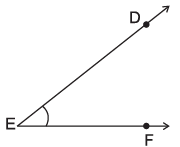Ans. Angle construction.

Q.6. Draw a line segment AB of length 10 cm. By drawing its perpendicular bisector repeatedly, obtain a line segment of length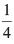AB.

Ans.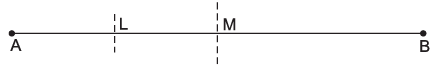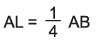Q.7. Draw a circle with centre X and radius 4 cm. Draw any chord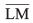Construct the perpendicular bnisector ofand check whether it passes through X. (Yes/No).

Ans. Yes and construction.

Q.8. Draw a line segment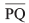of length 7 cm. Take any point, M, not on it. Through M, draw a perpendicular to, using ruler and compasses.

Ans. Construction.

Q.9. Draw a line segment AB of length 8 cm. At each end point of this line segment, draw a line perpendicular to AB. Are these two lines parallel? (Yes/No)

Ans. Construction, Yes.

Q.10. If AB = 7.5 cm and CD = 1.5 cm, construct a line segment whose length is equal to
(i) 2 CD
(ii) AB + CD
(iii) AB – CD

Ans. Construction.

The document Worksheet Question: Practical Geometry - Notes | Study Mathematics (Maths) Class 6 - Class 6 is a part of the Class 6 Course Mathematics (Maths) Class 6.
All you need of Class 6 at this link: Class 6

## Mathematics (Maths) Class 6

168 videos|282 docs|43 tests
 Use Code STAYHOME200 and get INR 200 additional OFF

## Mathematics (Maths) Class 6

168 videos|282 docs|43 tests

Track your progress, build streaks, highlight & save important lessons and more!

,

,

,

,

,

,

,

,

,

,

,

,

,

,

,

,

,

,

,

,

,

;